ChemTalk

# What is Enthalpy?## Core Concepts

In this tutorial, you will learn about the definition and equation of enthalpy. In addition, you will learn about some of its applications, as it relates to thermodynamics.

## Enthalpy Definition

Enthalpy (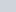) has to do with thermodynamics; it is a state function, at constant pressure, used in chemical and biological systems. This means that enthalpy depends only on the final energy, pressure, and volume and not the path the system took to get to the final state.

This is the change in internal energy that is equal to the heat transfer within the system. This is due to the law of energy conservation.

### Enthalpy Formula

This property is the sum of a system’s internal energy and the product of Pressure x Volume.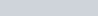: Enthalpy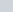: Internal energy of the system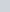: Pressure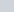: Volume

You also may see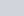expressed as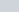, known as work.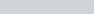The SI unit of measurement for enthalpy is the Joule (J). However, sometimes you may see the units of calorie or British thermal unit (BTU).

### Temperature Dependence

A factor that comes into play when determining enthalpy, even though it is not seen in the equations above, is temperature. The same reactants in a reaction can vary in the amount of heat they can transfer when at different temperatures. Additionally, different reactants may be in different phases of matter at different ambient temperatures, which can also play a role in heat transfer.

### Specific Enthalpy

Specific enthalpy,, is seen when doing calculations having to do with energy balancing; this is because, when looking at energy balancing equations, we are thinking about the difference in enthalpy in two different conditions, the change.

Usually, a reference value is given, whether from a table or presented in a word problem, in order to gauge the energy difference in the initial and final system conditions. Due to the fact thatcannot absolutely determined, it is likely to assign a reference state as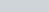. For example, when looking at water and water vapor, thevalue at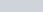and normal atmospheric pressure is assigned to be 0.

### Endothermic vs Exothermic

When a process is endothermic, it absorbs heat into the system. This causes the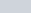value to be positive. On the other hand, when a process is exothermic, it releases heat into the environment. Remember that despite there seeming to be a change in energy, these reactions, like all chemical reactions, obey the law of conservation of energy.

### Entropy vs Enthalpy

With all the terms used in thermodynamics, it can be confusing to keep track of them all. Entropy (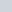) is the amount of disorder and randomness seen in a system. Simply, the more disorganized a reaction turns into, the more entropy there is; for example, a reaction going from solid to liquid has high entropy while a reaction going from gas to liquid has low entropy. This property can be further analyzed with topics such as Gibbs Free Energy.

## Applications of Enthalpy

There are chemical reactions going on everywhere in the real world, and there are various examples of enthalpy seen in everyday life. One example is portable hand warmer packets. These tiny packets undergo an iron oxidation reaction under constant pressure in a closed system, which is why when you break in the packet, it gets warm quickly and easily. This is an example of an exothermic reaction because energy is being released from the system in the form of heat. Another example of enthalpy can be seen in refrigerator compressors. Here, there is a vaporization reaction occurring, again under the exact condition of constant pressure. Energy is being used and absorbed in the system in the form of heat as the refrigerant chemicals are vaporized in an endothermic matter.

## Enthalpy Practice Problems

Problem 1

When water vapor condenses into liquid, heat is released into the environment. Is condensation, therefore, exothermic or endothermic?

Problem 2

Consider the equation for change in Gibbs free energy: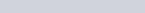If a certain chemical reaction involves a negative change in entropy (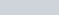) and a negative change in Gibbs free energy (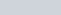), is it exothermic or endothermic? (Hint: since temperature is expressed in Kelvin, we know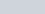)

1: Exothermic

2: Exothermic